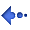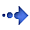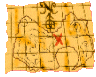Water Rockets - An explanation of equations

The water rocket computer model allows you to look at what the rocket will be doing at any particular point in time, during its flight. So, why do we need something as complicated as a computer model? Won't "normal" equations do? Do we have to use equations at all? Why isn't there just a table of pressures, weights and heights achieved? Before any of this is answered, we have to know what the limitations of equations are and before that, we need to know how an equation is put together. The following is an example of how an equation can be "built".

Supposing we need to find out the sum of a series of consecutive whole numbers starting with 1.

eg 1 + 2 + 3 = 6   or   1 + 2 + 3 + 4 = 10

If the sums are small, then a simple table would do where "n" is the highest number so, when the sum is 1 + 2 + 3 + 4,   n = 4 . . .

 n Sum 2 3 4 5 3 6 10 15

This is okay if n stays small but supposing n equalled 1037 (ie 1 + 2 + 3 + 4 . . . 1035 + 1036 + 1037). Clearly, a solution has to be found that allows us to work it out without having to resort to tables. Adding all of these numbers together is repetative addition as is multiplication. If we could convert the process into multiplication then we could save a lot of time and be able to work out the sum of any series in only a few presses of a calculator keypad.

Consider 1 + 2 + 3 + 4,   (n = 4)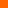This is the same as 4 + 3 + 2 + 1 (they both add up to 10)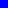If we put them together, we get (using brackets to make things clearer) . . .
(1 + 2 + 3 + 4) + (4 + 3 + 2 + 1)+Supposing we put the first number of the first set with the first number of the second set and so on . . .
(1 + 4) + (2 + 3) + (3 + 2) + (4 + 1)+++The total for each bracket is 5 and there are four of them. In other words, we have . . .
4 x (5). . . a simple multiplication. But this is twice the number that we need so if we divide by 2, we get the right number.
 4 x (5)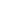2
Now, we need to turn it into an equation.
We said before that n = 4,  so  5 = n + 1

From that, we can say that . . .

 The sum of a series of whole numbers from 1 to n = n x (n + 1)2

Applying it to our problem of n = 1037,   the sum = 1037 + 1038 / 2 = 538203

Twelve keystrokes instead of 4,079, a few seconds work as opposed to over 1 hour of typing plus being confident that there are no mistakes (type it all in again to make sure???)

So, that is one way of making an equation. The sum of integers problem is an easy one as it is linear (it follows a straight line - it forms a rectangle and you can draw a straight line along the transition between the two sets of blocks). Sometimes, more complicated curves have to fit and sometimes the equation only allows an estimate.

The humble water rocket's flight characteristics do not go in a straight line:

• The thrust occurs over a period of time at the begining and it is not over in an instant so equations that have the rocket travelling at full speed at the beginning of the flight do not apply;
• The drag increases with the square of the speed;
• The drag always slows the rocket down, therefore on the way up, it is pulling the rocket downwards whereas on the way down, it is pulling the rocket upwards;
• The thrust changes during the burn, decreasing as the air pressure falls;
• The mass of the rocket plus the water changes during the burn as the water is expelled so the effects of the force of the thrust change - decreasing and then, maybe increasing as the burn proceeds;
• The pressure at the nozzle increases with acceleration;
• The pressure at the nozzle decreases as the height of liquid in the rocket decreases during the burn;
• If you use a launch tube . . . and it is hollow . . .;
• The pressure at the nozzle increases with acceleration;
• Once the thrust has ended, the rocket is in freefall; and, so on.

It is clear that we are not going to end up with a simple equation - more likely a complicated mess of equations that are limited in the range of application (maybe only working for a certain size of bottle weighing a certain amount with a constant thrust), not reflecting the true water rocket and therefore not satisfactory at all.

The only practical solution to all of these problems is to use a time-sliced computer model that takes the situation at the beginning and works out what is going to happen next (only a thousandth of a second later) and then, taking that situation, works out what is going to happen next . . . until the rocket touches down. That is what happens in the water rocket computer model. All of the application of all of the equations is done for you so you can concentrate on what you are interested in, whether it is finding the best weight of a rocket for travelling the furthest, or finding the maximum acceleration so that you can design some piece of electronic hardware.Back to the Index Copyright 1994 - 2003 P.A.Grosse. All Rights Reserved